### Home > CALC > Chapter 8 > Lesson 8.2.1 > Problem8-67

8-67.
1. Find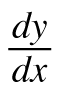for the following functions. Then evaluate the derivative at the given point. Homework Help ✎

1.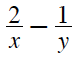= 1; (1,1)

2. x2y y3 = 8; (−3, 1)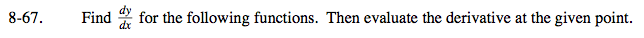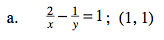$-2x^{-2}+y^{-2}y^\prime=0$

$-2(1)^{-2}+(1)^{-2}y^\prime=0$

Now solve for y.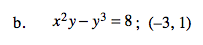$2xy+x^2y^\prime-3y^2y^\prime=0$